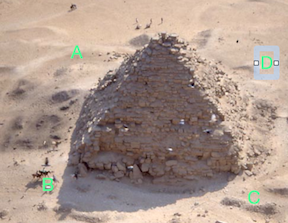# Galliv-Ant in Egypt

Geometry Level 3A right pyramid in Egypt has a square base $ABCD$ of $200 \text{ m}$ length and vertical height of $100 \text{ m}$. The minimum distance that an ant has to crawl to reach point $C$ from point$A$ is to the nearest $\text{ m}$ given by is?

×